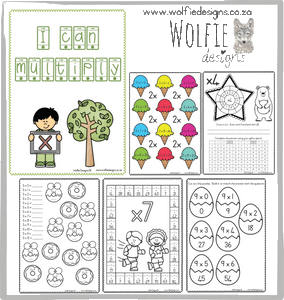# I can multiply

Regular price
R 25.00
Sale price
R 25.00
Regular price
R 60.00
Sold out
Unit price
per
Shipping calculated at checkout.

This book has 90 pages and consists of all the multiply tables from 1-12.

Every multiply tables consists of the following:

1.  A poster with the multiply table's questions and answers.

2.  6 Exercises where answers must be filled in.

3.  Flash cards with the multiply table's questions.

4.  Puzzles to match the multiply table's questions and answers.

These worksheets has a lot of pictures to make learning FUN!

Wolfie Designs is proud of QUALITY work!